_ ("blank") stands for anything. x_ ("x blank") stands for anything, but calls it x. _h stands for anything with head h. And x_h stands for anything with head h, and calls it x.
Define a function whose argument is an integer named n:
 In:=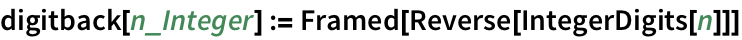The function evaluates whenever the argument is an integer:
 In:=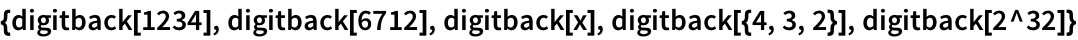Out=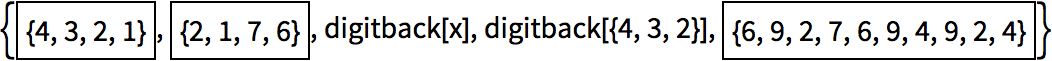Sometimes you may want to put a condition on a pattern. You can do this with /; (slash semi). n_Integer/;n>0 means any integer that is greater than 0.
Give a definition which only applies when n>0:
 In:=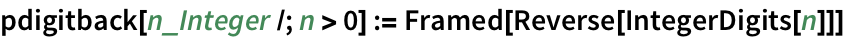The definition doesnt apply to negative numbers:
 In:=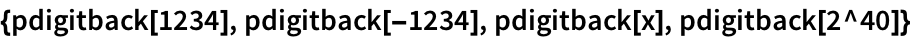Out=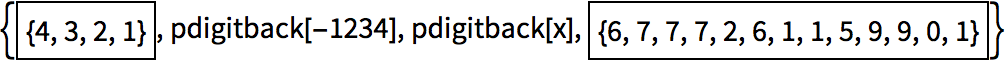The /; can go anywhereeven at the end of the whole definition.
Define different cases of the check function:
 In:=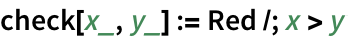In:=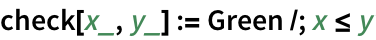Some examples of the check function:
 In:=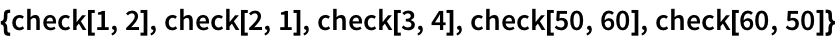Out=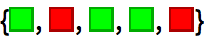__ (double blank) stands for any sequence of one or more arguments. ___ (triple blank) stands for zero or more.
Define a function that looks for black and white (in that order) in a list.
The pattern matches black followed by white, with any elements before, between and after them:
 In:=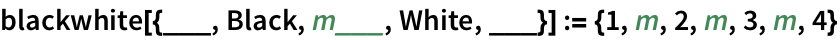In:=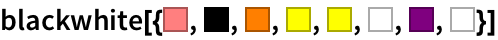Out=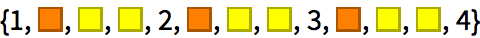By default, __ and ___ pick the shortest matches that work. You can use Longest to make them pick the longest instead.
Specify that the sequence between black and white should be as long as possible:
 In:=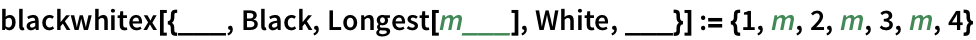Now m grabs elements all the way to the last white:
 In:=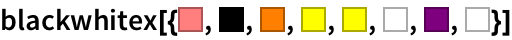Out=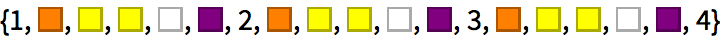x|y|z matches x, y or z. x.. matches any number of repetitions of x.
bwcut effectively cuts out the longest run containing only black and white:
 In:=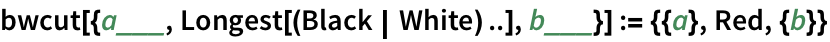In:=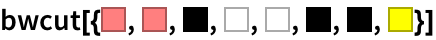Out=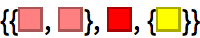The pattern x_ is actually short for x:_, which means match anything (i.e. ) and name the result x. You can use notations like x: for more complicated patterns too.
Set up a pattern named m that matches a list of two pairs:
 In:=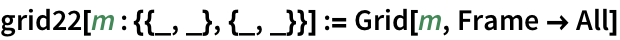In:=Out=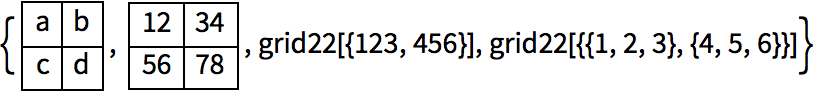Name the sequence of black and white, so it can be used in the result:
 In:=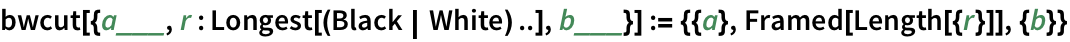In:=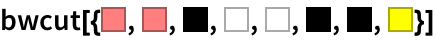Out=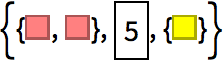Replace the first elements one finds out of order by ones that are in order:
 In:=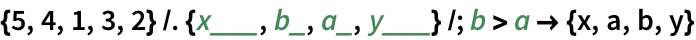Out=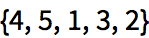In:=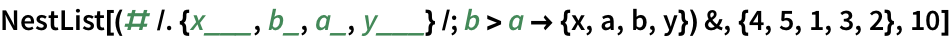Out=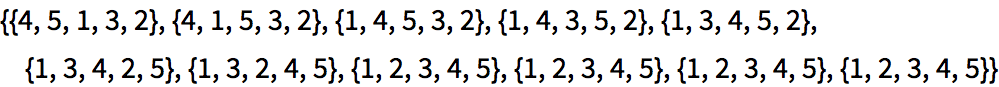At the beginning, we wont know how long itll take to finish sorting a particular list. So the best thing is to use FixedPointList, which is like NestList, except that you dont have to tell it a specific number of steps, and instead it just goes on until the result reaches a fixed point, where nothing more is changing.
 In:=Out=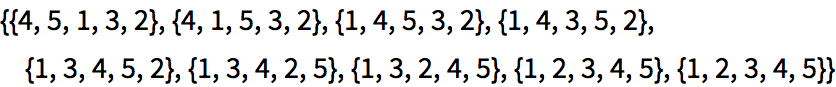In:=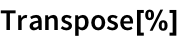Out=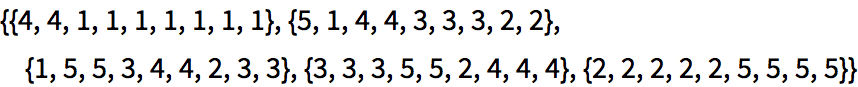ListLinePlot plots each list in a different color, showing how the sorting process proceeds:
 In:=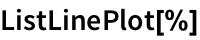Out=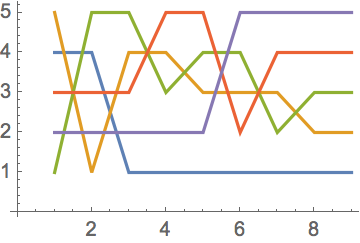Heres the result for sorting a random length-20 list:
 In:=Out=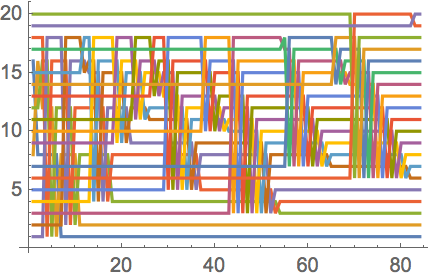patt/;cond a pattern that matches if a condition is met ___ a pattern for any sequence of zero or more elements (“triple blank”) patt.. a pattern for one or more repeats of patt Longest[patt] a pattern that picks out the longest sequence that matches FixedPointList[f,x] keep nesting f until the result no longer changes
41.1Find the list of digits for squares of numbers less than 100 that contain successive repeated digits. »
Expected output:
 Out[]=Answer & check your solution
41.2In the first 100 Roman numerals, find those containing L, I and X in that order. »
Expected output:
 Out[]=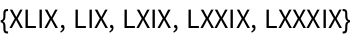Answer & check your solution
41.3Define a function f that tests whether a list of integers is the same as its reverse. »
No expected output
Many possible solutions of the form _:=_ or _=_Answer & check your solution
41.4Get a list of pairs of successive words in the Wikipedia article on alliteration that have identical first letters. »
Sample expected output:
 Out[]=Answer & check your solution
41.5Use Grid to show the sorting process in this section for {4, 5, 1, 3, 2}, with successive steps going down the page. »
Expected output:
 Out[]=Answer & check your solution
41.6Use ArrayPlot to show the sorting process in this section for a list of length 50, with successive steps going across the page. »
Sample expected output:
 Out[]=Answer & check your solution
Expected output:
 Out[]=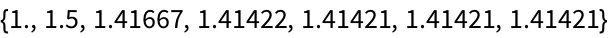Answer & check your solution
41.8Implement Euclids algorithm for GCD in which {a, b} is repeatedly replaced by {b, Mod[a, b]} until b is 0, and apply the algorithm to 12345, 54321»
Expected output:
 Out[]=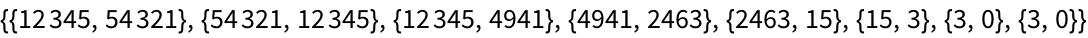Answer & check your solution
41.9Define combinators using the rules s[x_][y_][z_]x[z][y[z]], k[x_][y_]x, then generate a list by starting with s[s][k][s[s[s]][s]][s] and applying these rules until nothing changes. »
Expected output:
 Out[]=Answer & check your solution
41.10Remove all trailing 0s from the digit list for 100!»
Expected output:
 Out[]=Answer & check your solution
Expected output:
 Out[]=Answer & check your solution
Expected output:
 Out[]=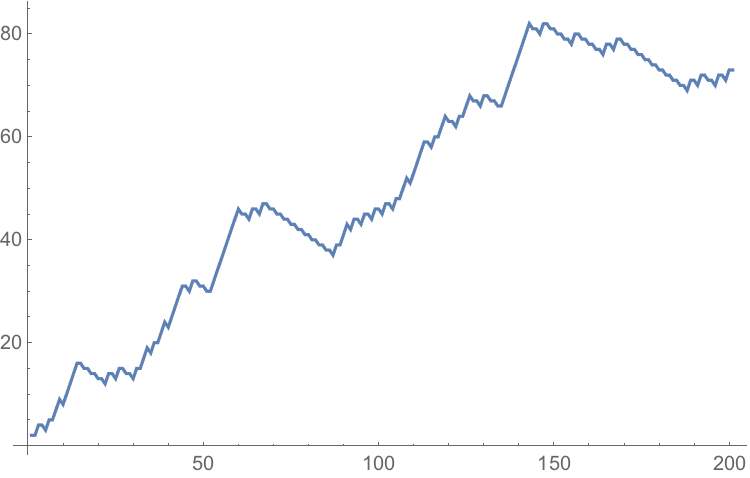Answer & check your solution
What are other pattern constructs in the Wolfram Language?
Except[patt] matches anything except patt. PatternSequence[patt] matches a sequence of arguments in a function. OrderlessPatternSequence[patt] matches them in any order. f[x_:v] defines v as a default value, so f[ ] is matched, with x being v.
How can one see all the ways a pattern could match a particular expression?
Use ReplaceList. Replace gives the first match; ReplaceList gives a list of all of them.
What does FixedPointList do if theres no fixed point?
Itll eventually stop. Theres an option that tells it how far to go. FixedPointList[f, x, n] stops after at most n steps.